1582458600

# Generating Poetic Texts with Recurrent Neural Networks in Python

In this tutorial we are creating our first AI project. It is a recurrent neural network that generates poetic texts, similar to those of Shakespeare!

## Introduction

Recurrent neural networks are very useful when it comes to the processing of sequential data like text. In this tutorial, we are going to use LSTM neural networks (Long-Short-Term Memory) in order to tech our computer to write texts like Shakespeare.

Yes you heard that right! We are going to train a neural network to write texts similar to those of the famous poet. At least kind of. Since recurrent neural networks and LSTMs in particular have a short term memory, we can train it to “guess” the next letter based on the letters that came before. That leads to our network producing these texts. For this of course we will need some substantial training data.

In this tutorial we will only need a Python installation and the libraries Tensorflow, NumPy and Random. Nothing else!

``````import random
import numpy as np
import tensorflow as tf

``````

Now we can get to work. First of all we need a large amount of sentences and texts from Shakespeare himself, in order to train the model. For this we will use this file! And we will download it directly into our script.

``````
filepath = tf.keras.utils.get_file('shakespeare.txt',

``````

Here we directly download the file into our script and start reading and decoding it. The next step is to prepare the data so that we can process it.

## Preparing Data

The problem that we have right now with our data is that we are dealing with text. We cannot just train a neural network on letters or sentences. We need to convert all of these values into numerical data. So we have to come up with a system that allows us to convert the text into numbers, then predict specific numbers based on that data and then again convert the resulting numbers back into text.

``````text = open(filepath, 'rb').read().decode(encoding='utf-8').lower()

``````

For the sake of simplicity I am going to modify the last code line that we wrote. In this case I immediately convert all of the text into lower-case so that we have fewer possible choices. Also I am not going to use the whole text file as training data. If you have the capacities or the time to train your model on the whole data, do it! It will produce much better results. But if your machine is slow or you have limited time, you might consider just using a part of the text.

``````text = text[300000:800000]

``````

Here we select all the characters from character number 300,000 up until 800,000. So we are processing a total of 500,000 characters, which should be enough for pretty descent results.

``````
characters = sorted(set(text))

char_to_index = dict((c, i) for i, c in enumerate(characters))
index_to_char = dict((i, c) for i, c in enumerate(characters))

``````

Now we create a sorted set of all the unique characters that occur in the text. In a set no value appears more than once, so this is a good way to filter out the characters. After that we define two structures for converting the values. Both are dictionaries that enumerate the characters. In the first one, the characters are the keys and the indices are the values. In the second one it is the other way around. Now we can easily convert a character into a unique numerical representation and vice versa.

``````SEQ_LENGTH = 40
STEP_SIZE = 3

sentences = []
next_char = []

``````

In this next step, we define how long a sequence shall be and also how many characters we will step further to start the next sentence. What we try to do here is to take sentences and then save the next letter as the training data.

``````
for i in range(0, len(text) - SEQ_LENGTH, STEP_SIZE):
sentences.append(text[i: i + SEQ_LENGTH])
next_char.append(text[i + SEQ_LENGTH])

``````

We iterate through the whole text and gather all sentences and their next character. This is the training data for our neural network. Now we just need to convert it into a numerical format.

``````
x = np.zeros((len(sentences), SEQ_LENGTH,
len(characters)), dtype=np.bool)
y = np.zeros((len(sentences),
len(characters)), dtype=np.bool)

for i, satz in enumerate(sentences):
for t, char in enumerate(satz):
x[i, t, char_to_index[char]] = 1
y[i, char_to_index[next_char[i]]] = 1

``````

This might seem a little bit complicated right now but it is not. We are creating two NumPy arrays full of zeros. The data type of those is bool, which stands for boolean. Wherever a character appears in a certain sentence at a certain position we will set it to a one or a True. We have one dimension for the sentences, one dimension for the positions of the characters within the sentences and one dimension to specify which character is at this position.

## Building Recurrent Neural Network

Now that our training data is prepared, let us start with building the neural network. To make our code simpler, we are going to import the specific tools that we are going to use.

``````
import random
import numpy as np
import tensorflow as tf
from tensorflow.keras.models import Sequential
from tensorflow.keras.optimizers import RMSprop
from tensorflow.keras.layers import Activation, Dense, LSTM

``````

Of course you can also just refer to these things manually if you want to. We will use Sequential for our model, Activation, Dense and LSTM for our layers and RMSprop for optimization during the compilation of our model. LSTM stands for long-short-term memory and is a type of recurrent neural network layer. It might be called the memory of our model. This is crucial, since we are dealing with sequential data.

``````
model = Sequential()
input_shape=(SEQ_LENGTH,
len(characters))))

``````

Our structure is simple! The inputs immediately flow into our LSTM layer with 128 neurons. Our input shape is the length of a sentence times the amount of characters. The character which shall follow will be set to True or one. This layer is followed by a Dense hidden layer, which just increases complexity. In the end we use the Softmax activation function in order to make our results add up to one. This gives us the probability for each character.

``````
model.compile(loss='categorical_crossentropy',
optimizer=RMSprop(lr=0.01))

model.fit(x, y, batch_size=256, epochs=4)

``````

Now we compile the model and train it with our training data that we prepared above. We choose a batch size of 256 (which you can change if you want) and four epochs. This means that our model is going to see the same data four times.

## Helper Function

Our model is now trained but it only outputs the probabilities for the next character. We therefore need some additional functions to make our script generate some reasonable text.

``````
def sample(preds, temperature=1.0):
preds = np.asarray(preds).astype('float64')
preds = np.log(preds) / temperature
exp_preds = np.exp(preds)
preds = exp_preds / np.sum(exp_preds)
probas = np.random.multinomial(1, preds, 1)
return np.argmax(probas)

``````

This helper function called sample is copied from the official Keras tutorial.

It basically just picks one of the characters from the output. As parameters it takes the result of the prediction and a temperature. This temperature indicates how risky the pick shall be. If we have a high temperature, we will pick one of the less likely characters. A low temperature will cause a conservative choice.

## Generating Text

Now we can get to the final function of our script. The function that generates the final text.

``````
def generate_text(length, temperature):
start_index = random.randint(0, len(text) - SEQ_LENGTH - 1)
generated = ''
sentence = text[start_index: start_index + SEQ_LENGTH]
generated += sentence
for i in range(length):
x_predictions = np.zeros((1, SEQ_LENGTH, len(characters)))
for t, char in enumerate(sentence):
x_predictions[0, t, char_to_index[char]] = 1

predictions = model.predict(x_predictions, verbose=0)
next_index = sample(predictions,
temperature)
next_character = index_to_char[next_index]

generated += next_character
sentence = sentence[1:] + next_character
return generated

``````

Again, it is less complicated than it looks. We basically choose a random starting position within the text because we need some starting text in order to predict the “next” character. So basically the first SEQ_LENGTH amount of characters will be copied from the original text. But we could just cut them off afterwards and we would end up with text that is completely generated by our neural network.

So we choose some random starting text and then we run a for loop in the range of the length that we want. We can generate a text with 100 characters or one with 20,000. We then convert our sentence into the desired input format that we already talked about. The sentence is now an array with ones or Trues, wherever a character occurs. Then we use the predict method of our model, to predict the likelihoods of the next characters. Then we make use of our sample helper function. In this function we also have a temperature parameter, which we can pass to that helper function. Of course the result we get needs to be converted from the numerical format into a readable character. Once this is done, we add the character to our generated text and repeat the process, until we reach the desired length.

## Results

The results are actually quite good! Let’s take a look at some samples. I played around with the parameters, in order to diversify the results. I am not going to post the full results here but just some interesting snippets.

``````
print(generate_text(300, 0.2))
print(generate_text(300, 0.4))
print(generate_text(300, 0.5))
print(generate_text(300, 0.6))
print(generate_text(300, 0.7))
print(generate_text(300, 0.8))

``````

Settings – Length: 300 – Temperature: 0.4

``````
ay, marry, thou dost the more thou dost the mornish,
and if the heart of she gentleman, or will,
the heart with the serving a to stay thee,
i will be my seek of the sould stay stay
the fair thou meanter of the crown of manguar;
the send their souls to the will to the breath:
the wry the sencing with the sen

``````

Settings – Length: 300 – Temperature: 0.6

``````
warwick:
and, thou nast the hearth by the createred
to the daughter, that he word the great enrome;
that what then; if thou discheak, sir.

clown:
sir i have hath prance it beheart like!

``````

Settings – Length: 300 – Temperature: 0.8

``````

i hear him speak.
what! can so young a thordo, word appeal thee,
but they go prife with recones, i thou dischidward!
has thy noman, lookly comparmal to had ester,
and, my seatiby bedath, romeo, thou lauke be;
how will i have so the gioly beget discal bone.

clown:
i have seemitious in the step--by this low,

``````

If you consider the fact that our computer doesn’t even understand what a word or a sentence is, these results are mind-blowing. Of course, they are not perfect and a lot of times (especially when choosing a high temperature), we will end up with some creative word creations. But still it is kind of impressive.

## Final Idea

That’s it for today’s tutorial. Of course, here we just used Shakespeare’s texts. You can also export your WhatsApp chats and load these into this script. If you want to see a tutorial on that, let me know in the comments!

I hope you enjoyed this blog post! If you want to tell me something or ask questions, feel free to ask in the comments! Down below you will find some additional links, including the full source code. Check out my Instagram page or the other parts of this website, if you are interested in more! I also have a lot of Python programming books! Stay tuned!

Originally published at neuralnine.com

#python #deep_learning #tensorflow

## Buddha Community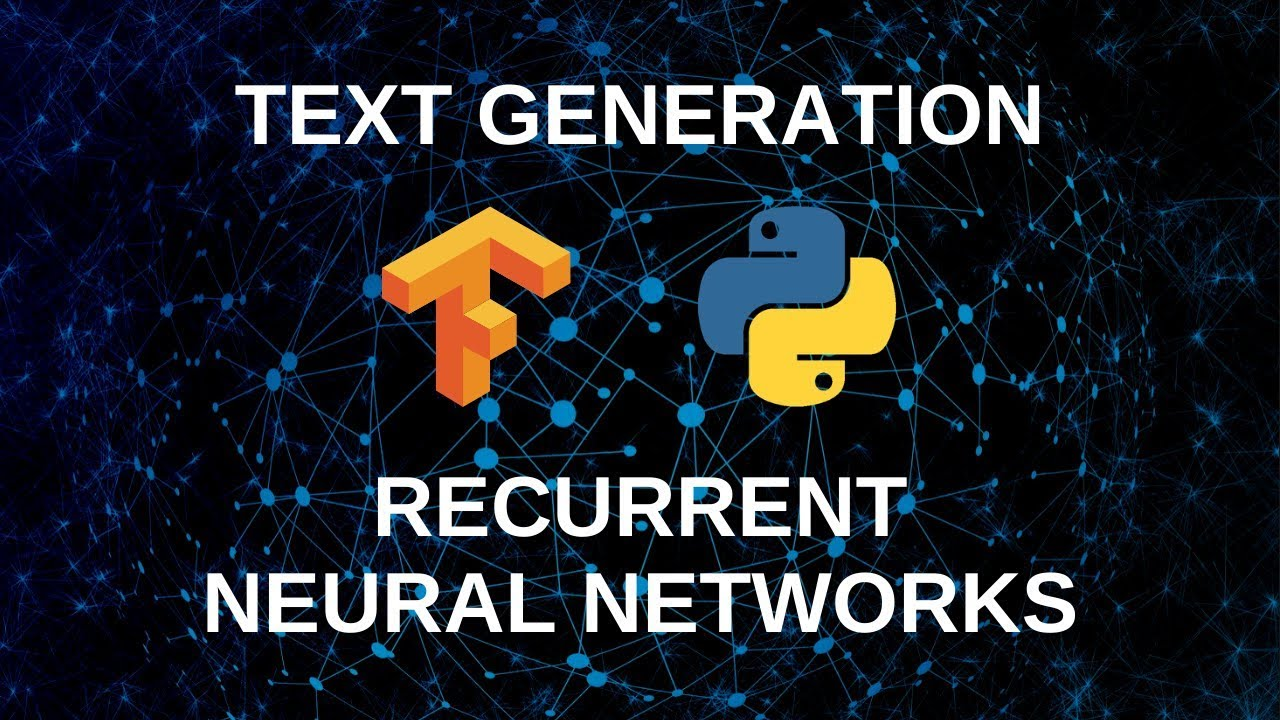1619518440

## top 30 Python Tips and Tricks for Beginners

Welcome to my Blog , In this article, you are going to learn the top 10 python tips and tricks.

### 8) Check The Memory Usage Of An Object.

#python #python hacks tricks #python learning tips #python programming tricks #python tips #python tips and tricks #python tips and tricks advanced #python tips and tricks for beginners #python tips tricks and techniques #python tutorial #tips and tricks in python #tips to learn python #top 30 python tips and tricks for beginners1619510796

## Lambda, Map, Filter functions in python

Welcome to my Blog, In this article, we will learn python lambda function, Map function, and filter function.

Lambda function in python: Lambda is a one line anonymous function and lambda takes any number of arguments but can only have one expression and python lambda syntax is

Syntax: x = lambda arguments : expression

Now i will show you some python lambda function examples:

#python #anonymous function python #filter function in python #lambda #lambda python 3 #map python #python filter #python filter lambda #python lambda #python lambda examples #python map1596825840

## A Comparative Analysis of Recurrent Neural Networks

Recurrent neural networks, also known as RNNs, are a class of neural networks that allow previous outputs to be used as inputs while having hidden states. RNN models are mostly used in the fields of natural language processing and speech recognition.

The vanishing and exploding gradient phenomena are often encountered in the context of RNNs. The reason why they happen is that it is difficult to capture long term dependencies because of multiplicative gradient that can be exponentially decreasing/increasing with respect to the number of layers.

Gated Recurrent Unit (GRU) and Long Short-Term Memory units (LSTM) deal with the vanishing gradient problem encountered by traditional RNNs, with LSTM being a generalization of GRU.

1D Convolution_ layer_ creates a convolution kernel that is convolved with the layer input over a single spatial (or temporal) dimension to produce a tensor of outputs. It is very effective for deriving features from a fixed-length segment of the overall dataset. A 1D CNN works well for natural language processing (NLP).

## DATASET: IMDb Movie Review

TensorFlow Datasets is a collection of datasets ready to use, with TensorFlow or other Python ML frameworks, such as Jax. All datasets are exposed as `[_tf.data.Datasets_](https://www.tensorflow.org/api_docs/python/tf/data/Dataset)`, enabling easy-to-use and high-performance input pipelines.

“imdb_reviews”

This is a dataset for binary sentiment classification containing substantially more data than previous benchmark datasets. It provides a set of 25,000 highly polar movie reviews for training, and 25,000 for testing.

## Import Libraries

``````import pandas as pd
import numpy as np
import matplotlib.pyplot as plt
import seaborn as sns
%matplotlib inline
``````

``````import tensorflow as tf
import tensorflow_datasets

imdb
``````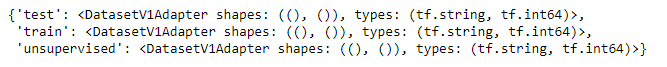``````info
``````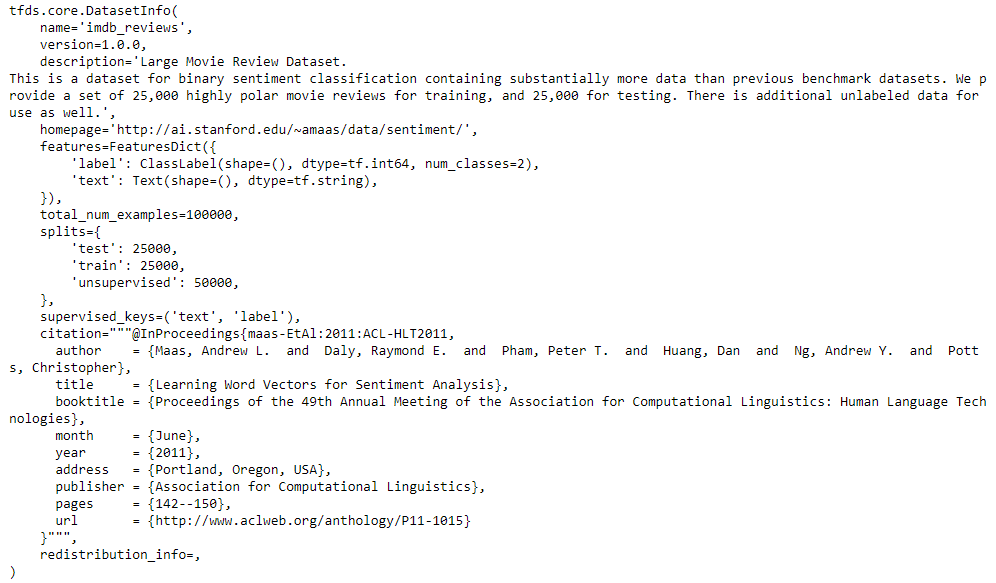## Training and Testing Data

``````train_data, test_data=imdb['train'], imdb['test']

training_sentences=[]
training_label=[]
testing_sentences=[]
testing_label=[]
for s,l in train_data:
training_sentences.append(str(s.numpy()))
training_label.append(l.numpy())
for s,l in test_data:
testing_sentences.append(str(s.numpy()))
testing_label.append(l.numpy())
training_label_final=np.array(training_label)
testing_label_final=np.array(testing_label)
``````

``````vocab_size=10000
embedding_dim=16
max_length=120
trunc_type='post'
oov_tok='<oov>'
from tensorflow.keras.preprocessing.text import Tokenizer
tokenizer= Tokenizer(num_words=vocab_size, oov_token=oov_tok)
tokenizer.fit_on_texts(training_sentences)
word_index=tokenizer.word_index
sequences=tokenizer.texts_to_sequences(training_sentences)
testing_sequences=tokenizer.texts_to_sequences(testing_sentences)
from tensorflow.keras.models import Sequential
from tensorflow.keras.layers import Dense, Dropout, Embedding
``````

## Multi-layer Bidirectional LSTM

#imdb #convolutional-network #long-short-term-memory #recurrent-neural-network #gated-recurrent-unit #neural networks1594366200

## Recurrent Neural Networks for Multilabel Text Classification Tasks

The purpose of this project is to build and evaluate Recurrent Neural Networks(RNNs) for sentence-level classification tasks. I evaluate three architectures: a two-layer Long Short-Term Memory Network(LSTM), a two-layer Bidirectional Long Short-Term Memory Network(BiLSTM), and a two-layer BiLSTM with a word-level attention layer. Although they do learn useful vector representation, BiLSTM with attention mechanism focuses on necessary tokens when learning text representation. To that end, I’m using the 2019 Google Jigsaw published dataset on Kaggle labeled “Jigsaw Unintended Bias in Toxicity Classification.” The dataset includes 1,804,874 user comments, with the toxicity level being between 0 and 1. The final models can be used for filtering online posts and comments, social media policing, and user education.

## Recurrent Neural Networks Overview

RNNs are neural networks used for problems that require sequential data processing. For instance:

• In a sentiment analysis task, a text’s sentiment can be inferred from a sequence of words or characters.
• In a stock prediction task, current stock prices can be inferred from a sequence of past stock prices.

At each time step of the input sequence, RNNs compute the output yt and an internal state update ht using the input xt and the previous hidden-state ht-1. They then pass information about the current time step of the network to the next. The hidden-state ht summarizes the task-relevant aspect of the past sequence of the input up to t, allowing for information to persist over time.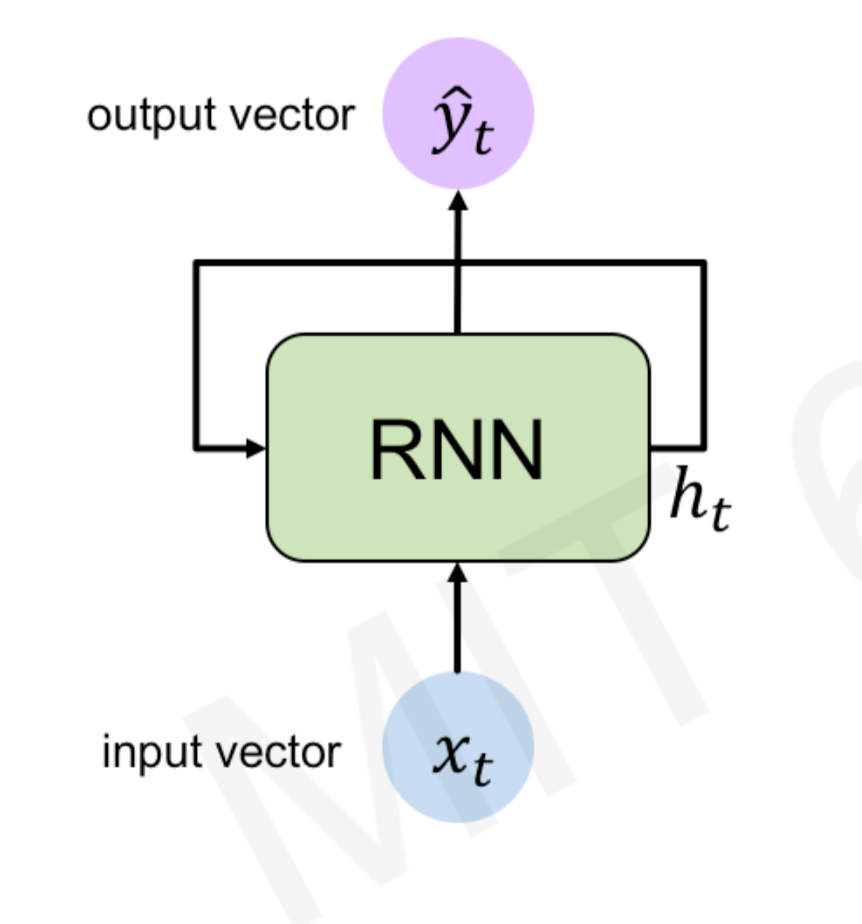Recurrent Neural Network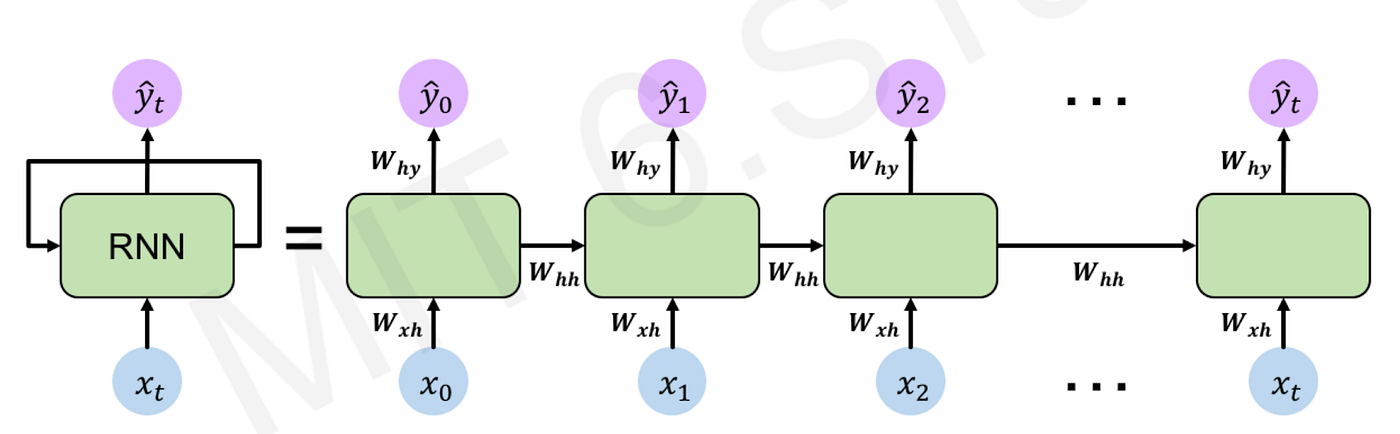Recurrent Neural Network

During training, RNNs re-use the same weight matrices at each time step. Parameter sharing enables the network to generalize to different sequence lengths. The total loss is a sum of all losses at each time step, the gradients with respect to the weights are the sum of the gradients at each time step, and the parameters are updated to minimize the loss function.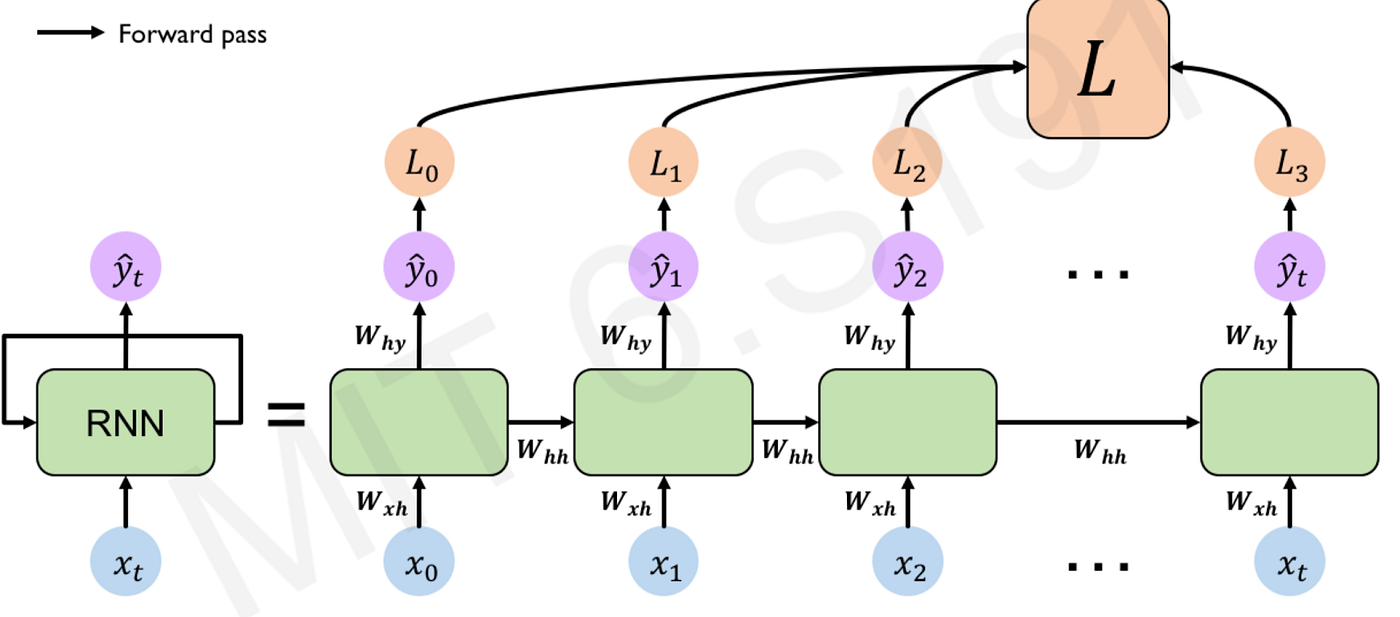forward pass: compute the loss function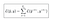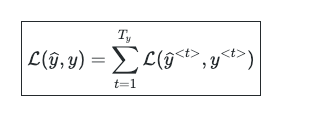loss function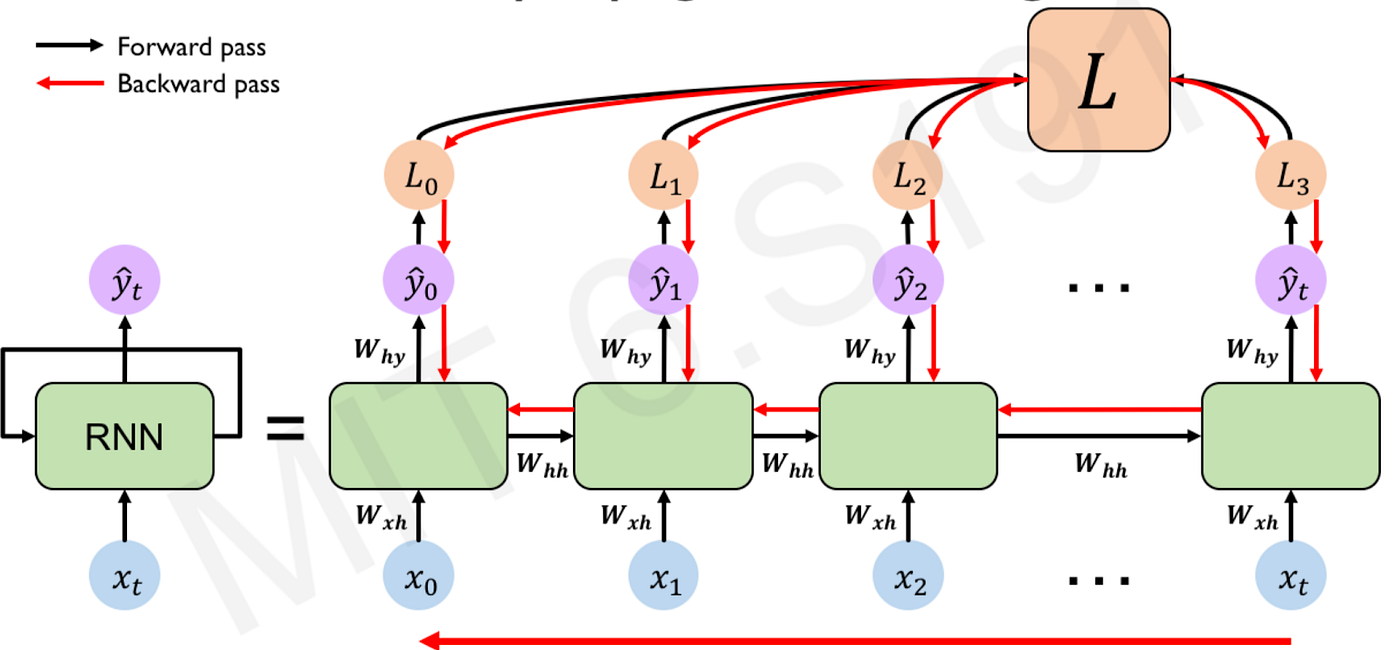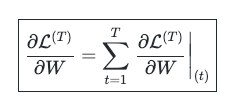Although RNNs learn contextual representations of sequential data, they suffer from the exploding and vanishing gradient phenomena in long sequences. These problems occur due to the multiplicative gradient that can exponentially increase or decrease through time. RNNs commonly use three activation functions: RELU, Tanh, and Sigmoid. Because the gradient calculation also involves the gradient with respect to the non-linear activations, architectures that use a RELU activation can suffer from the exploding gradient problem. Architectures that use Tanh/Sigmoid can suffer from the vanishing gradient problem. Gradient clipping — limiting the gradient within a specific range — can be used to remedy the exploding gradient. However, for the vanishing gradient problem, a more complex recurrent unit with gates such as Gated Recurrent Unit (GRU) or Long Short-Term Memory (LSTM) can be used.

#ai #recurrent-neural-network #attention-network #machine-learning #neural-network1599758100

## Convert Text to Speech in Python

Learn how to convert your Text into Voice with Python and Google APIs

Text to speech is a process to convert any text into voice. Text to speech project takes words on digital devices and convert them into audio with a button click or finger touch. Text to speech python project is very helpful for people who are struggling with reading.

#### Project Prerequisites

To implement this project, we will use the basic concepts of Python, Tkinter, gTTS, and playsound libraries.

• Tkinter is a standard GUI Python library that is one of the fastest and easiest ways to build GUI applications using Tkinter.
• gTTS (Google Text-to-Speech) is a Python library, which is a very easy library that converts the text into audio.
• The playsound module is used to play audio files. With this module, we can play a sound file with a single line of code.

To install the required libraries, you can use pip install command:

``````pip install tkinter
pip install gTTS
pip install playsound
``````

#### Text to Speech Python Project

The objective of this project is to convert the text into voice with the click of a button. This project will be developed using Tkinter, gTTs, and playsound library.

In this project, we add a message which we want to convert into voice and click on play button to play the voice of that text message.

• Importing the modules
• Create the display window
• Define functions

So these are the basic steps that we will do in this Python project. Let’s start.

#python tutorials #python project #python project for beginners #python text to speech #text to speech convertor #python